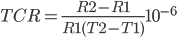# Temperature Coefficient of Resistance

## Resistance changes with temperature

The temperature coefficient of resistance, or TCR, is one of the main used parameters to characterize a resistor. The TCR defines the change in resistance as a function of the ambient temperature. The common way to express the TCR is in ppm/°C, which stands for parts per million per centigrade degree. The temperature coefficient of resistance is calculated as follows:Where TCR is in ppm/°C, R1 is in ohms at room temperature, R2 is resistance at operating temperature in ohms, T1 is the room temperature in °C and T2 is the operating temperature in °C. Often instead of TCR, α is used.Average TCR ΔR/R in ppm for a temperature range of -55 till 25˚C and 25 till 125˚C

## Positive or Negative Temperature Coefficient of Resistance?

Resistors are available with a TCR that is negative, positive, or stable over a certain temperature range. Choosing the right resistor could prevent the need for temperature compensation. In some applications it is desired to have a large TCR, for example to measure temperature. Resistors for these applications are called thermistors, and can have a positive (PTC) or negative temperature coefficient (NTC).

## Measuring methods for the TCR

The temperature coefficient of resistance for a resistor is determined by measuring the resistances values over an appropriate temperature range. The TCR is calculated as the average slope of the resistance value over this interval. This is accurate for linear relations, since the TCR is constant at every temperature. However, many materials have a non linear coefficient. For Nichrome for example, a popular alloy for resistors, the relation between temperature and TCR is not linear. Because the TCR is calculated as average slope, it is therefore very important to specify the TCR as well as the temperature interval. The way to measure TCR is standardized in MIL-STD-202 Method 304. With this method, TCR is calculated for the range between -55°C and 25°C and between 25°C and 125°C. Because the highest measured value is defined as TCR, this method often results in over specifying a resistor for less demanding applications.

In the chart below the temperature coefficient of resistance is given for a wide variety of materials. Note that the exact value depends on the purity of the material as well as the temperature.

 Material TCR /°C Silicon -0.075 Germanium -0.048 Carbon (amorphous) -0.0005 Manganin 0.000002 Constantan 0.000008 Nichrome 0.0004 Mercury 0.0009 Gold 0.0034 Zinc 0.0037 Copper 0.0039 Aluminum 0.0039 Lead 0.0039 Platinum 0.00392 Calcium 0.0041 Tungsten 0.0045 Tin 0.0045 Iron 0.005 Nickel 0.006 Lithium 0.006

Values for TCR depend on purity and temperature.

• Share